Next: Structure identification Up: Learning with Mixtures of Previous: Decomposable priors for tree

Experiments

This section describes the experiments that were run in order to assess the promise of the MT model. The first experiments are structure identification experiments; they examine the ability of the MIXTREE algorithm to recover the original distribution when the data are generated by a mixture of trees. The next group of experiments studies the performance of the MT model as a density estimator; the data used in these experiments are not generated by mixtures of trees. Finally, we perform classification experiments, studying both the MT model and a single tree model. Comparisons are made with classifiers trained in both supervised and unsupervised mode. The section ends with a discussion of the single tree classifier and its feature selection properties. In all of the experiments the training algorithm is initialized at random, independently of the data. Unless stated otherwise, the learning algorithm is run until convergence. Log-likelihoods are expressed in bits/example and therefore are sometimes called compression rates. The lower the value of the compression rate, the better the fit to the data. In the experiments that involve small data sets we use the Bayesian methods that we discussed in Section 4 to impose a penalty on complex models. In order to regularize model structure we use a decomposable prior over tree edges with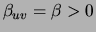. To regularize model parameters we use a Dirichlet prior derived from the pairwise marginal distributions for the data set. This approach is known as smoothing with the marginal [Friedman, Geiger, Goldszmidt 1997,Ney, Essen, Kneser 1994]. In particular, we set the parameter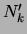characterizing the Dirichlet prior for tree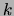by apportioning a fixed smoothing coefficient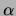equally between the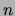variables and in an amount that is inversely proportional to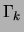between the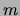mixture components. Intuitively, the effect of this operation is to make thetrees more similar to each other, thereby reducing the effective model complexity.

SubsectionsNext: Structure identification Up: Learning with Mixtures of Previous: Decomposable priors for tree
Journal of Machine Learning Research 2000-10-19